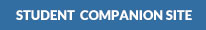Lent:

Learning to Program with MATLAB: Building GUI Tools, 2nd Edition•Codes From Textbook (requires WinZip or equivalent software)

•Codes From Textbook (requires WinZip or equivalent software)

•Codes From Textbook (requires WinZip or equivalent software)

•Codes From Textbook (requires WinZip or equivalent software)

•Codes From Textbook (requires WinZip or equivalent software)

•Codes From Textbook (requires WinZip or equivalent software)

•Codes From Textbook (requires WinZip or equivalent software)

•Codes From Textbook (requires WinZip or equivalent software)

•Codes From Textbook (requires WinZip or equivalent software)

•Codes From Textbook (requires WinZip or equivalent software)

•Codes From Textbook (requires WinZip or equivalent software)

•Codes From Textbook (requires WinZip or equivalent software)

## Get Help With:

* These links will open a new window